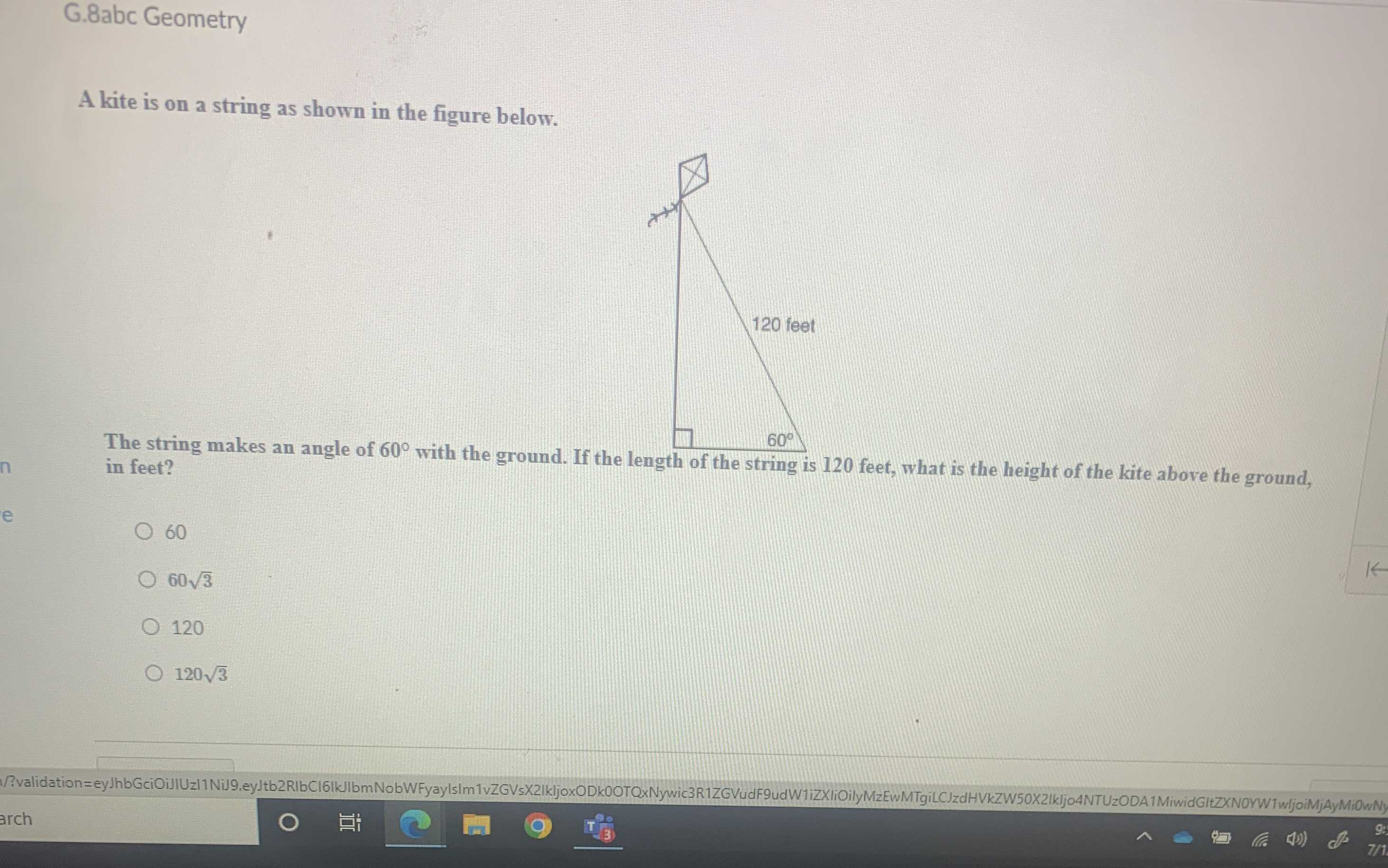### Still have math questions?

Trigonometry
QuestionA kite is on a string as shown in the figure below.

The string makes an angle of $$60 ^ { \circ }$$ with the ground. If the length of the string is $$120$$ feet, what is the height of the kite above the ground, in feet?

$$60$$

$$60 \sqrt { 3 }$$

$$120$$

$$120 \sqrt { 3 }$$

60$$\sqrt { 3}$$